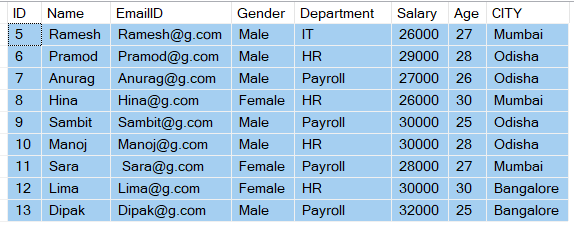# Comparison Operators in SQL Server

##### Comparison Operators in SQL Server with Examples

In this article, I am going to discuss the Comparison Operators in SQL Server with Examples. Please read our previous article where we discussed the Arithmetic Operators with examples. At the end of this article, you will understand what are comparison operators and their use in SQL server and different type of comparison operators like equal (=), greater than (>), less than (<), greater than or equal to (>=), less than or equal to (<=), not equal (<>), etc. in detail with examples.

##### What are Comparison Operators in SQL Server?

The comparison operators in SQL Server are used to compare two values. That means comparison operators are used to comparing one expression with another expression. The result of a comparison can be TRUE, FALSE, or UNKNOWN (an operator that has one or two NULL expressions returns UNKNOWN).

Note: You can use comparison operators on all the expressions except expressions of type text, ntext, or image.

##### Types of Comparison Operators in SQL Server

The different types of comparison operators that are available in SQL Server are as follows:

1. Equal (=) Operator
2. Not Equal (!= or <>) Operator
3. Greater Than (>) Operator
4. Less Than (<) Operator
5. Greater Than or Equal To (>=) Operator
6. Less Than or Equal To (<=) Operator
7. Not Greater Than (!<) Operator
8. Not Less Than (!>) Operator
##### Understanding the Comparison Operators in SQL Server with Examples:

Let us understand how to use the comparison operators with some examples. We are going to use the following Employee table to understand these operators.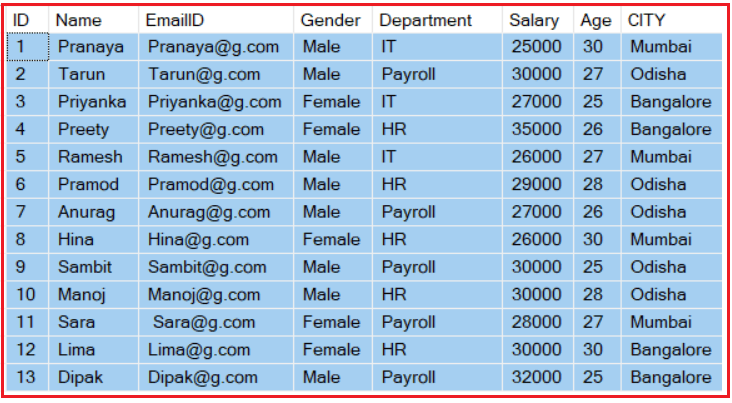Please use the below script to create the database EmployeeDB, Employee table, and populate Employee table with the test data.

```--Create database EmployeeDB
Create database EmployeeDB
Go

Use EmployeeDB
Go

-- Create Employee table
CREATE TABLE Employee
(
ID INT PRIMARY KEY IDENTITY(1,1),
Name VARCHAR(100),
EmailID VARCHAR(100),
Gender VARCHAR(100),
Department VARCHAR(100),
Salary INT,
Age INT,
CITY VARCHAR(100)
)
GO

--Insert some test data into Employee table
INSERT INTO Employee VALUES('Pranaya','Pranaya@g.com','Male', 'IT', 25000, 30,'Mumbai')
INSERT INTO Employee VALUES('Tarun','Tarun@g.com','Male', 'Payroll', 30000, 27,'Odisha')
INSERT INTO Employee VALUES('Priyanka','Priyanka@g.com','Female', 'IT', 27000, 25,'Bangalore')
INSERT INTO Employee VALUES('Preety','Preety@g.com','Female', 'HR', 35000, 26,'Bangalore')
INSERT INTO Employee VALUES('Ramesh','Ramesh@g.com','Male','IT', 26000, 27,'Mumbai')
INSERT INTO Employee VALUES('Pramod','Pramod@g.com','Male','HR', 29000, 28,'Odisha')
INSERT INTO Employee VALUES('Anurag','Anurag@g.com','Male', 'Payroll', 27000, 26,'Odisha')
INSERT INTO Employee VALUES('Hina','Hina@g.com','Female','HR', 26000, 30,'Mumbai')
INSERT INTO Employee VALUES('Sambit','Sambit@g.com','Male','Payroll', 30000, 25,'Odisha')
INSERT INTO Employee VALUES('Manoj','Manoj@g.com','Male','HR', 30000, 28,'Odisha')
INSERT INTO Employee VALUES('Sara',' Sara@g.com','Female', 'Payroll', 28000, 27,'Mumbai')
INSERT INTO Employee VALUES('Lima','Lima@g.com','Female','HR', 30000, 30,'Bangalore')
INSERT INTO Employee VALUES('Dipak','Dipak@g.com','Male','Payroll', 32000, 25,'Bangalore')
GO
```
##### Equal (=) Operator in SQL Server

The equal (=) operator in SQL Server is used to check whether the two expressions are equal or not. If it’s equal then the condition becomes true and it will return the matched records. For example, the below SQL statement will return records from the Employee table where the CITY is Mumbai.

SELECT * FROM Employee WHERE CITY = ‘Mumbai’

Once you execute the above query, you will get the following output.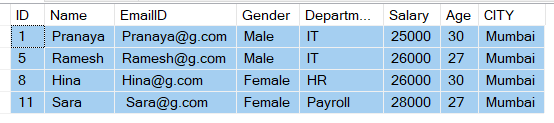###### Not Equal (!=) Operator in SQL Server

This is the opposite of the equal operator. That means the not equal (!=) operator is used to check whether the two expressions are equal or not. If it’s not equal then the condition becomes true and it will return the not-matched records. For example, the following SQL query will return all records from the Employee table except the employees whose CITY is Mumbai.

SELECT * FROM Employee WHERE CITY != ‘Mumbai’

Once you execute the above SQL query, you will get the following output.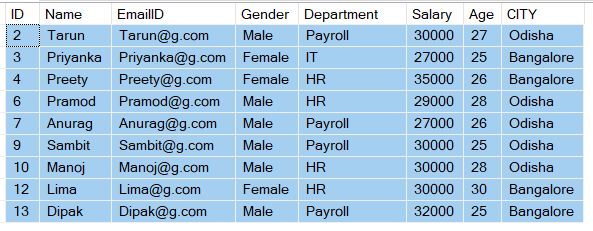###### Not Equal (<>) Operator in SQL Server

The not equal (<>) operator in SQL Server is used to check whether the two expressions are equal or not. If it’s not equal then the condition becomes true and it will return the not-matched records. The example is given below.

SELECT * FROM Employee WHERE CITY <> ‘Mumbai’

Following is the output of the above SQL query.Note Both != and <> operators are not equal operators and will return the same result but != operator is not an ISO standard.

##### Greater Than (>) Operator in SQL Server

The Greater Than (>) operator in SQL Server is used to check whether the left-hand operator is higher than the right-hand operator or not. If the left-hand operator higher than the right-hand operator then the condition becomes true and it will return the matched records. The following SQL query will return records from the Employee table where the ID is greater than 5.

SELECT * FROM Employee WHERE ID > 5

Following is the output of the above SQL query.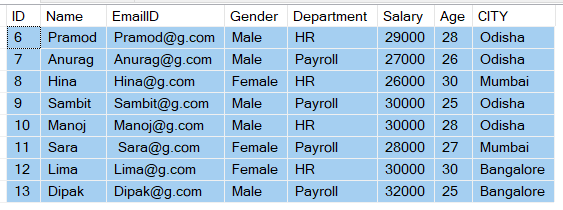##### Less Than (<) Operator in SQL Server

The less than (<) operator in SQL Server is used to check whether the left-hand operator is lower than the right-hand operator or not. If the left-hand operator lower than the right-hand operator then the condition becomes true and it will return the matched records. The below SQL statement will return records from the Employee table where the ID is less than 5.

SELECT * FROM Employee WHERE ID < 5

Following is the output of the above SQL query.##### Greater Than or Equal To (>=) Operator in SQL Server

The Greater than or Equal To (>=) operator in SQL Server is used to check whether the left-hand operator is higher than or equals to the right-hand operator or not. If the left-hand operator is higher than or equals to the right-hand operator then the condition becomes true and it will return the matched records. The following SQL statement will return records from the Employee table where the Salary is greater than or equal to 29000.

SELECT * FROM Employee WHERE Salary >= 29000

Following is the output of the above SQL query.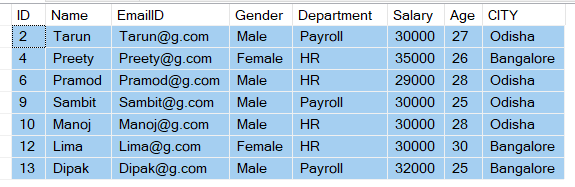##### Less Than or Equal To (<=) Operator in SQL Server

The Less Than or Equal To (<=) operator in SQL Server is used to check whether the left-hand operator is lower than or equals to the right-hand operator or not. If the left-hand operator is lower than or equals to the right-hand operator then the condition becomes true and it will return the matched records. The below SQL query will return records from the Employee table where the Salary is less than or equal to 29000.

SELECT * FROM Employee WHERE Salary <= 29000

Following is the output of the above SQL query.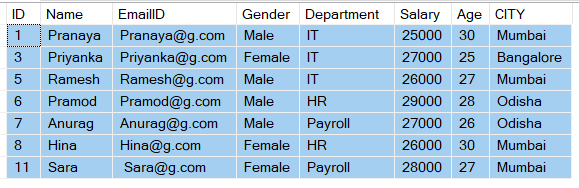##### Not Greater Than (!>) Operator in SQL Server

The Not Greater Than (!>) operator in SQL Server is used to check whether the left-hand operator is not greater than the right-hand operator or not. If the left-hand operator is not greater than the right-hand operator then the condition becomes true and it will return the matched records. The following SQL statement will return records from the Employee table where the employee ID is not greater than 5.

SELECT * FROM Employee WHERE ID !> 5

Following is the output of the above SQL query.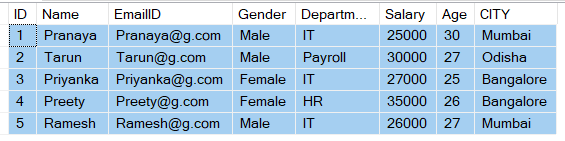##### Not Less Than (!<) Operator in SQL Server

The Not Less Than (!<) operator in SQL Server is used to check whether the left-hand operator is not less than the right-hand operator or not. If the left-hand operator is not less than the right-hand operator then the condition becomes true and it will return the matched records. The below SQL query will return records from the Employee table where the employee ID is not less than 5.

SELECT * FROM Employee WHERE ID !< 5

Following is the output of the above SQL query.